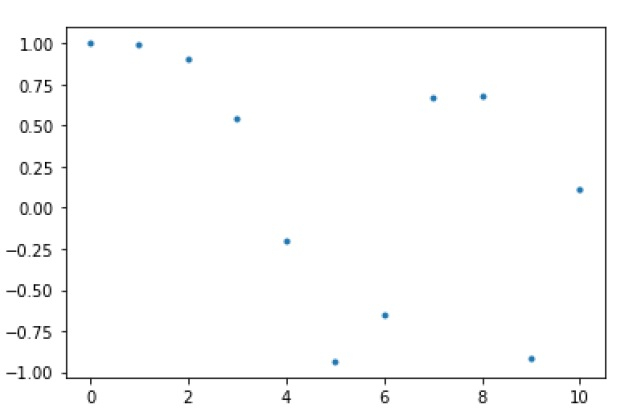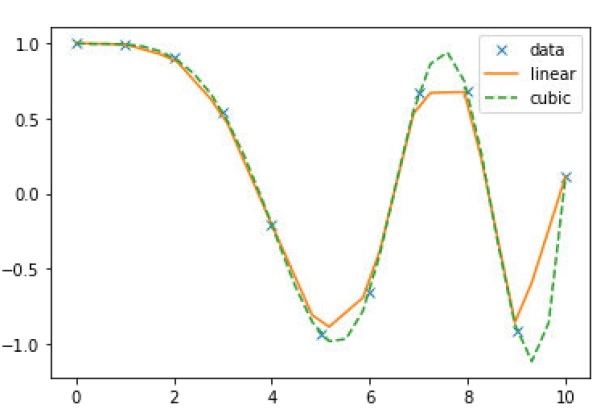# Comparing ‚Äòcubic‚Äô and ‚Äòlinear‚Äô 1-D interpolation using SciPy library

Below python script will compare the ‘cubic’ and ‘linear’ interpolation on same data using SciPy library −

## Example

First let’s generate some data to implement interpolation on that −

import numpy as np
from scipy.interpolate import interp1d
import matplotlib.pyplot as plt
A = np.linspace(0, 10, num=11, endpoint=True)
B = np.cos(-A**2/9.0)
print (A, B)

## Output

The above script will generate the following points between 0 and 4 −

[ 0. 1. 2. 3. 4. 5. 6. 7. 8. 9. 10.] [ 1. 0.99383351 0.90284967 0.54030231 -0.20550672 -0.93454613 -0.65364362 0.6683999 0.67640492 -0.91113026 0.11527995]

Now, let’s plot these points as follows −

plt.plot(A, B, '.')
plt.show()Now, based on fixed data points, we need to create two interpolate functions-one for ‘linear’ and other for ‘cubic’. Let’s create it −

function_interpolate1 = interp1d(A, B, kind = 'linear')
function_interpolate2 = interp1d(A, B, kind = 'cubic')

To see the clear difference in both interpolations, we will create a new input of more length by using the same function as used for old input −

Anew = np.linspace(0, 10, num=30, endpoint=True)
plt.plot(A, B, 'x', Anew, function_interpolate1(Anew), '-',
Anew,function_interpolate2(Anew), '--')
plt.legend(['data', 'linear', 'cubic'], loc = 'best')
plt.show()